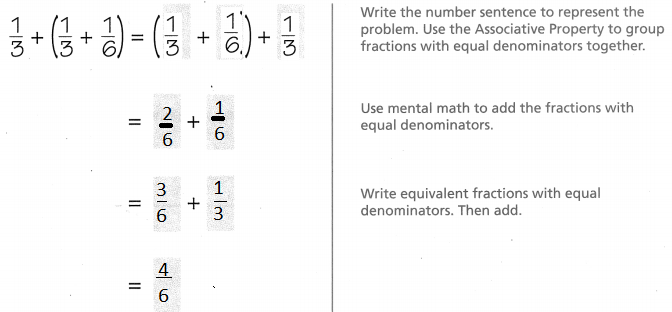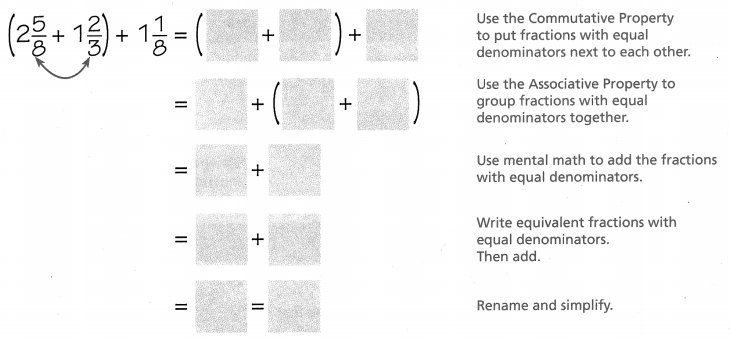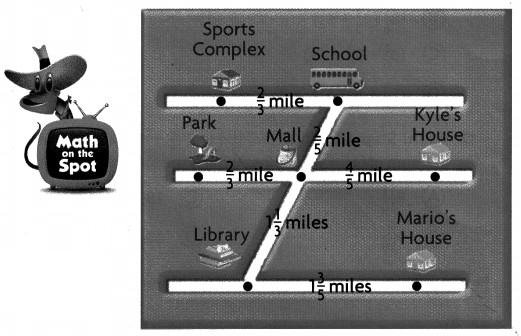Refer to our Texas Go Math Grade 5 Answer Key Pdf to score good marks in the exams. Test yourself by practicing the problems from Texas Go Math Grade 5 Lesson 5.8 Answer Key Use Properties of Addition.

Unlock the Problem

Jane and her family are driving to Big Lagoon State Park. On the first day, they travel $$\frac{1}{3}$$ of the total distance. On the second day, they travel $$\frac{1}{3}$$ of the total distance in the morning and then $$\frac{1}{6}$$ of the total distance in the afternoon. How much of the total distance has Jane’s family driven by the end of the second day?

Use the Associative Property

Day 1 + Day 2So, Jane’s family has driven _________ of the total distance by the end of the second day.So, Jane’s family has driven $$\frac{4}{6}$$ of the total distance by the end of the second day.

Math Talk
Mathematical Processes

Explain Why grouping the fractions differently makes it easier to find the sum.
Because, Adding 2 fraction makes very tough
so, grouping the fractions differently makes it easier to find the sum.

Example

Use the Commutative Property and the Associative Property.Try This!

Subtraction is not commutative or associative. When you subtract, perform operations in parentheses first. Then subtract from left to right.
a. $$\frac{7}{8}$$ – $$\frac{1}{2}$$ – $$\frac{1}{8}$$ = __________ – $$\frac{1}{8}$$ = ____________
$$\frac{7}{8}$$ – $$\frac{1}{2}$$ – $$\frac{1}{8}$$ =$$\frac{3}{8}$$ – $$\frac{1}{8}$$ = $$\frac{2}{8}$$

b. ($$\frac{7}{8}$$ – $$\frac{1}{2}$$) – $$\frac{1}{8}$$ = ____________ – $$\frac{1}{8}$$ = ____________
($$\frac{7}{8}$$ – $$\frac{1}{2}$$) – $$\frac{1}{8}$$ = ____________ – $$\frac{1}{8}$$ = ____________

c. $$\frac{7}{8}$$ – ($$\frac{1}{2}$$ – $$\frac{1}{8}$$) = $$\frac{3}{8}$$ –$$\frac{1}{8}$$= $$\frac{2}{8}$$
($$\frac{7}{8}$$ – $$\frac{1}{2}$$) – $$\frac{1}{8}$$ = $$\frac{7}{8}$$ – $$\frac{1}{8}$$ = $$\frac{6}{8}$$

Explain how you can use your answers to conclude that subtraction is not associative.
The equation one and two shows that by using associative property addition gives the same answer
but two and three shows that by using associative property subtraction gives the different answer

Share and Show

Use the properties and mental math to solve. Write your answer in the simplest form.

Question 1.
(2$$\frac{5}{8}$$ + $$\frac{5}{6}$$) + 1$$\frac{1}{8}$$
($$\frac{21}{8}$$ + $$\frac{5}{6}$$) + $$\frac{9}{8}$$
($$\frac{63}{24}$$ + $$\frac{40}{24}$$) + $$\frac{27}{8}$$
$$\frac{103}{24}$$ =
$$\frac{130}{24}$$
Explanation:
Written the number sentence to represent the problem.
Used the Associative Property to group fractions with equal denominators together.
Used mental math to add the fractions with
equal denominators.
Written equivalent fractions with equal denominators and then added

Question 2.
$$\frac{5}{12}$$ + ($$\frac{5}{12}$$ + $$\frac{3}{4}$$)
($$\frac{5}{12}$$ + $$\frac{9}{12}$$) + $$\frac{5}{12}$$
$$\frac{5+5+9}{12}$$ =
$$\frac{19}{12}$$
Explanation:
Written the number sentence to represent the problem.
Used the Associative Property to group fractions with equal denominators together.
Used mental math to add the fractions with
equal denominators.
Written equivalent fractions with equal denominators and then added

Question 3.
(3$$\frac{1}{4}$$ + 2$$\frac{5}{6}$$) + 1$$\frac{3}{4}$$
($$\frac{39}{12}$$ + $$\frac{34}{12}$$) + $$\frac{7}{4}$$
$$\frac{94}{12}$$
Explanation:
Written the number sentence to represent the problem.
Used the Associative Property to group fractions with equal denominators together.
Used mental math to add the fractions with
equal denominators.
Written equivalent fractions with equal denominators and then added

Problem Solving

Use the properties and mental math to solve. Write your answer in simplest form.

Question 4.
($$\frac{2}{7}$$ + $$\frac{1}{3}$$) + $$\frac{2}{3}$$
($$\frac{2}{7}$$ + $$\frac{1}{3}$$) + $$\frac{2}{3}$$
($$\frac{6}{21}$$ + $$\frac{7}{21}$$) + $$\frac{2}{3}$$
($$\frac{13}{21}$$ + $$\frac{2}{3}$$)
$$\frac{13 +14}{21}$$ =
$$\frac{27}{21}$$
Explanation:
Written the number sentence to represent the problem.
Used the Associative Property to group fractions with equal denominators together.
Used mental math to add the fractions with
equal denominators.
Written equivalent fractions with equal denominators and then added

Question 5.
($$\frac{1}{5}$$ + $$\frac{1}{2}$$) + $$\frac{2}{5}$$
($$\frac{1}{5}$$ + $$\frac{1}{2}$$) + $$\frac{2}{5}$$
($$\frac{2}{10}$$ + $$\frac{5}{10}$$) + $$\frac{4}{10}$$
$$\frac{11}{10}$$
Explanation:
Written the number sentence to represent the problem.
Used the Associative Property to group fractions with equal denominators together.
Used mental math to add the fractions with
equal denominators.
Written equivalent fractions with equal denominators and then added

Question 6.
($$\frac{1}{6}$$ + $$\frac{3}{7}$$) + $$\frac{2}{7}$$
($$\frac{1}{6}$$ + $$\frac{3}{7}$$) + $$\frac{2}{7}$$
($$\frac{7}{42}$$ + $$\frac{24}{42}$$) + $$\frac{18}{42}$$
$$\frac{49}{42}$$
Explanation:
Written the number sentence to represent the problem.
Used the Associative Property to group fractions with equal denominators together.
Used mental math to add the fractions with
equal denominators.
Written equivalent fractions with equal denominators and then added

Question 7.
Explain why grouping the fractions differently makes it easier to find the sum.
Explanation:
Written the number sentence to represent the problem.
Used the Associative Property to group fractions with equal denominators together.
Used mental math to add the fractions with
equal denominators.
Written equivalent fractions with equal denominators and then added by this process it becomes easy

Problem Solving

Use the map to solve 8-9.

Question 8.
H.O.T. Multi-Step On one afternoon, Mario walks from his house to the library. That evening, Mario walks from the library to the mall, and then to Kyle’s house. Describe how you can use the properties to find how far Mario walks.($$\frac{8}{5}$$ + $$\frac{2}{5}$$) + $$\frac{4}{5}$$
$$\frac{8+2+4}{5}$$
$$\frac{14}{5}$$
Explanation:
Written the number sentence to represent the problem.
Used the Associative Property to group fractions with equal denominators together.
Used mental math to add the fractions with
equal denominators.
Written equivalent fractions with equal denominators and then added by this process it becomes easy

Question 9.
H.O.T. Pose a Problem Write and solve a new problem that uses the distances between four locations.
Answer: On one evening, Mario walks from his mall to the school. That evening, Mario walks from the school to the sports complex,  Describe how you can use the properties to find how far Mario walks.
($$\frac{2}{5}$$ + $$\frac{2}{3}$$)
$$\frac{6+10}{15}$$
$$\frac{16}{15}$$

Question 10.
During a scavenger hunt, Ben’s team completed four tasks in the following times: 2$$\frac{1}{3}$$ hours, 1$$\frac{1}{2}$$ hours, 1$$\frac{1}{3}$$ hours, and 1$$\frac{1}{3}$$ hours. How long did it take Ben’s team to complete the scavenger hunt?
(A) 6$$\frac{1}{2}$$ hours
(B) 5$$\frac{1}{2}$$ hours
(C) 6 hours
(D) 5 hours
2$$\frac{1}{3}$$ hours+1$$\frac{1}{2}$$ hours+ 1$$\frac{1}{3}$$ hours+1$$\frac{1}{3}$$ hours.
$$\frac{14+9+8+8}{6}$$ hours+$$\frac{39}{6}$$

Question 11.
Use Symbols Elijah wants to add (2$$\frac{3}{5}$$ + 8$$\frac{1}{6}$$) + 5$$\frac{1}{5}$$. He rewrites the problem as (8$$\frac{1}{6}$$ + 2$$\frac{3}{5}$$) + 5$$\frac{1}{5}$$. Then he uses the Associative Property to rewrite the problem. Which shows his next step?
(A) 8$$\frac{1}{6}$$ + (2$$\frac{3}{5}$$ + 5$$\frac{1}{5}$$)
(B) (8$$\frac{1}{6}$$) (2$$\frac{3}{5}$$ + 5$$\frac{1}{5}$$)
(C) (8$$\frac{1}{6}$$ + 2$$\frac{3}{5}$$ + 5$$\frac{1}{5}$$)
(D) (2$$\frac{3}{8}$$ + 8$$\frac{1}{6}$$) (5$$\frac{1}{5}$$)
Explanation:
His next step by using the property is
8$$\frac{1}{6}$$ + (2$$\frac{3}{5}$$ + 5$$\frac{1}{5}$$)

Question 12.
Multi-Step Glen finds the sum of (3$$\frac{3}{10}$$ + 4$$\frac{1}{3}$$) + 2$$\frac{1}{10}$$ and Ana finds the sum of (4$$\frac{1}{3}$$ + 3$$\frac{3}{10}$$) + 2$$\frac{1}{10}$$. What is the total sum of their answers?
(A) 9$$\frac{11}{15}$$
(B) 9$$\frac{1}{15}$$
(C) 18$$\frac{7}{15}$$
(D) 19$$\frac{7}{15}$$
Explanation:
9$$\frac{11}{15}$$ + 9$$\frac{11}{15}$$
19$$\frac{7}{15}$$

Texas Test Prep

Question 13.
Use the properties and mental math to solve.
$$\frac{11}{12}$$ – ($$\frac{2}{3}$$ – $$\frac{1}{12}$$)
(A) $$\frac{7}{12}$$
(B) $$\frac{1}{3}$$
(C) $$\frac{1}{4}$$
(D) $$\frac{1}{6}$$
Explanation:
$$\frac{11}{12}$$ – ($$\frac{2}{3}$$ – $$\frac{1}{12}$$)
$$\frac{1}{6}$$

### Texas Go Math Grade 5 Lesson 5.8 Homework and Practice Answer Key

Use the properties and mental math to solve. Write your answer in the simplest form.

Question 1.
($$\frac{3}{7}$$ + $$\frac{2}{3}$$) + $$\frac{1}{3}$$ ___________
($$\frac{3}{7}$$ + $$\frac{2}{3}$$) + $$\frac{1}{3}$$
($$\frac{9}{21}$$ + $$\frac{14}{21}$$) + $$\frac{1}{2}$$
($$\frac{23}{21}$$ + $$\frac{1}{2}$$)
$$\frac{23 + 21}{21}$$ =
$$\frac{44}{21}$$
Explanation:
Written the number sentence to represent the problem.
Used the Associative Property to group fractions with equal denominators together.
Used mental math to add the fractions with
equal denominators.
Written equivalent fractions with equal denominators and then added

Question 2.
$$\frac{4}{7}$$ + ($$\frac{1}{6}$$ + $$\frac{2}{7}$$) ____________
($$\frac{4}{7}$$ + $$\frac{1}{6}$$) + $$\frac{2}{7}$$
($$\frac{7}{42}$$ + $$\frac{2}{42}$$) + $$\frac{4}{7}$$
$$\frac{24 + 9}{42}$$ =
$$\frac{33}{42}$$
Explanation:
Written the number sentence to represent the problem.
Used the Associative Property to group fractions with equal denominators together.
Used mental math to add the fractions with
equal denominators.
Written equivalent fractions with equal denominators and then added

Question 3.
($$\frac{4}{5}$$ + $$\frac{1}{2}$$) + $$\frac{2}{5}$$ _____________
($$\frac{4}{5}$$ + $$\frac{1}{2}$$) + $$\frac{2}{5}$$
($$\frac{8 + 5}{10}$$ + $$\frac{2}{5}$$)
$$\frac{13 + 4}{10}$$ =
$$\frac{17}{10}$$
Explanation:
Written the number sentence to represent the problem.
Used the Associative Property to group fractions with equal denominators together.
Used mental math to add the fractions with
equal denominators.
Written equivalent fractions with equal denominators and then added

Question 4.
(3$$\frac{5}{8}$$ + $$\frac{1}{6}$$) + 2$$\frac{1}{8}$$ ____________
(3$$\frac{5}{8}$$ + $$\frac{1}{6}$$) + 2$$\frac{1}{8}$$
($$\frac{29}{8}$$ + $$\frac{1}{6}$$) + $$\frac{17}{8}$$
$$\frac{87 +51 + 4 }{24}$$ =
$$\frac{142}{24}$$
Explanation:
Written the number sentence to represent the problem.
Used the Associative Property to group fractions with equal denominators together.
Used mental math to add the fractions with
equal denominators.
Written equivalent fractions with equal denominators and then added

Question 5.
2$$\frac{1}{6}$$ + (2$$\frac{1}{4}$$ + 1$$\frac{1}{6}$$) ______________
2$$\frac{1}{6}$$ + (2$$\frac{1}{4}$$ + 1$$\frac{1}{6}$$)
($$\frac{9}{4}$$ + $$\frac{7}{6}$$) + $$\frac{13}{6}$$
$$\frac{26+27+14}{12}$$ =
$$\frac{67}{12}$$
Explanation:
Written the number sentence to represent the problem.
Used the Associative Property to group fractions with equal denominators together.
Used mental math to add the fractions with
equal denominators.
Written equivalent fractions with equal denominators and then added

Question 6.
($$\frac{1}{7}$$ + $$\frac{1}{6}$$) + $$\frac{3}{7}$$ ______________
($$\frac{1}{7}$$ + $$\frac{1}{6}$$) + $$\frac{3}{7}$$
($$\frac{6+7+18}{4}$$ = $$\frac{31}{42}$$)

Explanation:
Written the number sentence to represent the problem.
Used the Associative Property to group fractions with equal denominators together.
Used mental math to add the fractions with
equal denominators.
Written equivalent fractions with equal denominators and then added

Question 7.
($$\frac{3}{4}$$ + $$\frac{7}{12}$$) + $$\frac{5}{12}$$ ____________
($$\frac{3}{4}$$ + $$\frac{7}{12}$$) + $$\frac{5}{12}$$
($$\frac{9+7+5}{12}$$
$$\frac{21}{12}$$

Explanation:
Written the number sentence to represent the problem.
Used the Associative Property to group fractions with equal denominators together.
Used mental math to add the fractions with
equal denominators.
Written equivalent fractions with equal denominators and then added

Question 8.
$$\frac{3}{5}$$ + (2$$\frac{1}{5}$$ + 1$$\frac{1}{4}$$) ______________
$$\frac{3}{5}$$ + (2$$\frac{1}{5}$$ + 1$$\frac{1}{4}$$)
($$\frac{3}{5}$$ + $$\frac{11}{5}$$) + $$\frac{5}{4}$$
$$\frac{12+44+25}{20}$$ =
$$\frac{81}{20}$$
Explanation:
Written the number sentence to represent the problem.
Used the Associative Property to group fractions with equal denominators together.
Used mental math to add the fractions with
equal denominators.
Written equivalent fractions with equal denominators and then added

Question 9.
(3$$\frac{1}{2}$$ + 1$$\frac{3}{5}$$) + 1$$\frac{1}{2}$$ _____________
(3$$\frac{1}{2}$$ + 1$$\frac{3}{5}$$) + 1$$\frac{1}{2}$$
($$\frac{7}{2}$$ + $$\frac{8}{5}$$) + $$\frac{3}{2}$$
$$\frac{35+16+15}{10}$$ =
$$\frac{66}{10}$$
Explanation:
Written the number sentence to represent the problem.
Used the Associative Property to group fractions with equal denominators together.
Used mental math to add the fractions with
equal denominators.
Written equivalent fractions with equal denominators and then added

Question 10.
Write a word problem for the addends $$\frac{7}{10}$$, $$\frac{1}{5}$$ and $$\frac{9}{10}$$. Explain how you solved your problem.
$$\frac{7}{10}$$ + $$\frac{1}{5}$$ + $$\frac{9}{10}$$
$$\frac{7+2+9}{10}$$ =
$$\frac{18}{10}$$
Explanation:
Written the number sentence to represent the problem.
Used the Associative Property to group fractions with equal denominators together.
Used mental math to add the fractions with
equal denominators.
Written equivalent fractions with equal denominators and then added

Problem Solving

Question 11.
Last night, Halley spent $$\frac{4}{5}$$ hour on math homework, $$\frac{1}{2}$$ hour on science homework, and $$\frac{3}{5}$$ hour on social studies homework. How long did Halley spend on homework last night?
Answer: $$\frac{19}{10}$$
Explanation
$$\frac{4}{5}$$ + $$\frac{1}{2}$$ + $$\frac{3}{5}$$
$$\frac{8+5+6}{10}$$
$$\frac{19}{10}$$

Question 12.
The rainfall totals at the airport for the last three months were 2$$\frac{1}{6}$$ inches, 1$$\frac{2}{3}$$ inches, and 1$$\frac{1}{6}$$ inches. What was the total rainfall for the last three months?
Answer: $$\frac{90}{18}$$
Explanation:
2$$\frac{1}{6}$$ + 1$$\frac{2}{3}$$ +1$$\frac{1}{6}$$
$$\frac{39+30+21}{18}$$
$$\frac{90}{18}$$

Lesson Check

Question 13.
Shelly volunteered at the pet shelter 2$$\frac{1}{2}$$ hours in June, 1$$\frac{2}{3}$$ hours in July, and the same amount of hours in August as in June. How many hours did Shelly volunteer this summer?
(A) 4$$\frac{1}{6}$$ hours
(B) 5 hours
(C) 6$$\frac{2}{3}$$ hours
(D) 5$$\frac{2}{3}$$ hours
Explanation:
2$$\frac{1}{2}$$ + 2$$\frac{1}{2}$$ + 1$$\frac{2}{3}$$
$$\frac{40}{6}$$
6$$\frac{2}{3}$$ hours

Question 14.
Erica uses a recipe for cookies that calls for 2$$\frac{3}{4}$$ cups of flour and 1$$\frac{1}{2}$$ cups of sugar. Erica doubles the recipe and adds the flour and sugar to a mixing bowl. Which is the amount of dry ingredients in the bowl?
(A) 5$$\frac{3}{4}$$ cups
(B) 8$$\frac{1}{2}$$ cups
(C) 4$$\frac{1}{4}$$ cups
(D) 9$$\frac{1}{2}$$ cups
Explanation:
2$$\frac{3}{4}$$ + 1$$\frac{1}{2}$$
$$\frac{34}{8}$$ x 2
$$\frac{68}{2}$$
8$$\frac{1}{2}$$ cups

Question 15.
Stefan wants to add (3$$\frac{5}{7}$$ + 1$$\frac{1}{5}$$) + 2$$\frac{1}{7}$$. He uses the Commutative Property to rewrite the problem. Which shows this step?
(A) (3$$\frac{5}{7}$$ + 1$$\frac{1}{5}$$ + 2$$\frac{1}{7}$$)
(B) 3$$\frac{5}{7}$$ + (1$$\frac{1}{5}$$ + 2$$\frac{1}{7}$$)
(C) (1$$\frac{1}{5}$$ + 3$$\frac{5}{7}$$) + 2$$\frac{1}{7}$$
(D) (2$$\frac{1}{7}$$ + 1$$\frac{1}{5}$$) + 3$$\frac{5}{7}$$
(3$$\frac{5}{7}$$ + 1$$\frac{1}{5}$$) + 2$$\frac{1}{7}$$
3$$\frac{5}{7}$$ + (1$$\frac{1}{5}$$ + 2$$\frac{1}{7}$$)

Question 16.
Habib is making a frame for a rectangular picture that is 2$$\frac{1}{4}$$ feet by 3$$\frac{3}{8}$$ feet. How many feet of wood trim should Habib buy to make the frame?
(A) 6$$\frac{3}{4}$$ feet
(B) 10$$\frac{1}{4}$$ feet
(C) 11$$\frac{1}{4}$$ feet
(D) 5$$\frac{5}{7}$$ feet

Question 17.
Multi-Step Juliana used 10$$\frac{1}{2}$$ yards of yarn to make three yarn dolls. She used 4$$\frac{1}{2}$$ yards of yarn for the first doll and 2$$\frac{1}{5}$$ yards for the second doll. How much yarn did Juliana use for the third doll?
(A) 3$$\frac{4}{5}$$ yards
(B) 2$$\frac{4}{5}$$ yards
(C) 3$$\frac{1}{5}$$ yards
(D) 2$$\frac{1}{5}$$ yards
Explanation:
4$$\frac{1}{2}$$ + 2$$\frac{1}{5}$$
$$\frac{67}{10}$$ + 10$$\frac{1}{2}$$
3$$\frac{4}{5}$$ yards

Question 18.
Multi-Step Yuan finds the sum of $$\frac{3}{10}$$ + ($$\frac{1}{5}$$ + $$\frac{1}{10}$$). Then he adds $$\frac{1}{15}$$ to the sum. What is Yuan’s final sum?
(A) $$\frac{3}{5}$$
(B) $$\frac{1}{5}$$
(C) $$\frac{1}{3}$$
(D) $$\frac{2}{3}$$
$$\frac{3}{10}$$ + ($$\frac{1}{5}$$ + $$\frac{1}{10}$$)
$$\frac{3+2+1}{10}$$
$$\frac{6}{10}$$ + $$\frac{1}{15}$$
$$\frac{75}{150}$$
$$\frac{2}{3}$$